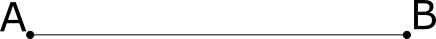Roughness Iteration Delay (ms) Iterations Displacement

The midpoint displacement algorithm is a simple algorithm to generate a fractal terrain. The algorithm can be extended to three dimensions, but the diamond-square algorithm is generally used instead. The algorithm works by repeated subdivisions of a line that are displaced by a random value, this random value is weighted by the recursion depth. This results in large displacement values for the first few subdivisions, which make up large hills on the terrain, followed by smaller displacements that help texture the terrain and make it look more realistic.

The algorithm works by the following process. Take a straight line, points A and B.Next, we divide the line into two equal parts (at point C) and move the y position of this newly generated point by a random factor with maximum displacement $D_{max}$We can scale the maximum value of the random generator by a weighting factor.

Here a roughness of 0 will result in the maximum displacement value, whereas a value of 1 will halve the maximum displacement, and thus generate a smoother terrain.

Now that we have updated the maximum displacement parameter, we continue the subdivision process. As there are now two divisions of the line, the next subdivision will result in four divisions in the line (at points D and E). The newly generated points on the line are again displaced by a random valueThis process is repeated until the desired recursion depth has been reached